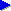HomeMemory managementJava equivalent to...constnewdeletemalloc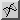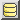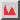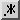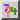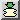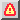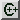# What is the Java equivalent of unsigned?

In C and C++, the unsigned modifier can be added to a integer variable declaration. It tells the compiler to treat the value of the variable as an unsigned value in arithmetic operations. Unsigned arithmetic is typically used:

• when manipulating raw bytes: it's often convenient to treat a byte as unsigned;
• when performing bitwise operations: that is, cases such as hashing or randum number generation, where the numerical value of the number doesn't actually have much sense, and we're just interested in performing bitwise operations such as XOR, shifts etc and interpreting the number as a "series of bits";
• where the sign makes no sense, and/or where we'd rather have a larger possible range of positive values than the possibility of negative numbers: such as a memory location or file size.

When an integer is signed, one of its bits becomes the sign bit, meaning that the maximum magnitude of the number is halved. (So an unsigned 32-bit int can store up to 232-1, whereas its signed counterpart has a maximum positive value of 231-1.)

In Java, all integer types are signed (except char). Although a questionable design, even bytes are signed in Java! So what do we do if we want to treat a value as unsigned in Java? In most typical cases in which unsigned values are used, it actually turns out not to be too difficult to get the same result in Java.

## Bitwise operations

It's important to remember that the unsigned keyword affects the interpretation, not the representation of a number. In other words, in cases where we aren't interpreting a value arithmetically— so-called bitwise operations such as AND, OR, XOR— it makes essentially no difference whether a value is marked as "signed" or "unsigned".

### Signed vs unsigned shifts

An important exception is the right shift operator, represented by two "greater than" symbols: >>. In both C/C++ and Java, this operator performs sign extension: that is, as well as shifting the bits of the number one place to the right, it preserves the sign. Specifically, after performing the shift, it copies the sign bit (the leftmost bit) into the leftmost position.

Now, if we're treating an integer as unsigned, then we don't want to copy the sign bit, because it doesn't actually represent the sign! Instead, we want to leave it as zero. To achieve this, in Java, instead of writing >>, we write >>>. This variant of the shift is sometimes called a logical shift, and the previous variant— which takes account of the sign— an arithmetic shift. At the machine code level, most architectures in fact provide different instructions for the two shifts, and the C/C++ compiler chooses the appropriate one depending on whether we've declared the variable in question as unsigned. In Java, we must explicitly say which type we require.

### Example: XORshift random number generator

As an example of unsigned bitwise operations in C/C++ vs Java, we'll look at the XORshift random number generator. Invented by Goerge Marsaglia (2003)1, the function provides a fast means of generating medium-quality random numbers using only a single variable or register. Each pass generates a new random number using two left shifts and one right shift, plus three exclusive or (XOR) operations, all using unsigned arithmetic. In C/C++, we simply declare the variable as unsigned. In Java, we ignore the signedness for the two bitwise operations (XOR and left shift) where it is irrelevant, and explicitly use signless logical shift right:

 C/C++: ```unsigned int seed = ...; int randNumber() { seed ^= (seed << 1); seed ^= (seed >> 5); // 2 > symbols! seed ^= (seed << 9); } ``` Java: ```int seed = ...; int randNumber() { seed ^= (seed << 1); seed ^= (seed >>> 5); // 3 > symbols! seed ^= (seed << 9); } ```

## Arithmetical operations

By arithmetical operations on unsigned integers, we mean cases where we want the upper bit to represent magnitude. Normally with a (signed) Java int, the result of the following would be a negative number, as we "roll over" past the largest positive integer that an int can store:

```int n = 1 << 31;
System.out.println("n was " + n);
n *= 2;
System.out.println("n is now " + n);
```

gives:

```n was 1073741824
n is now -2147483648
```

The usual way of getting round this problem is simply to use a type with a larger size and then "chop" off the extra bits (set them to zero). For example:

• to store an unsigned int, we would use a Java long;
• to store an unsigned byte, we could use any other integer type, but an int is generally convenient (it is likely to give faster arithmetic);
• if we want an unsigned long, we may be a bit stuck, although we could use a BigInteger object.

To "chop off the extra bits", we need to AND with the bits that we are interested in. For example, if we want to end up with an unsigned byte (8 bits), we need to AND with the value 0xff (255 in decimal)— 11111111 in binary or in other words, "the first 8 bits set". So the following are essentially equivalent:

 C/C++: ```unsigned byte b = ...; b += 100; unsigned int v = ...; v *= 2; ``` Java: ```int b = ...; b = (b + 100) & 0xff; long v = ...; v = (v * 2) & 0xffffffff; ```

When we eventually want to write the unsigned value, it is OK to simply cast to the appropriate size. For example:

```// Example: write an unsigned int (stored in a long)
// to a byte buffer.
ByteBuffer bb = ...
long unsignedInt = ...
bb.putInt((int) unsignedInt);
```

### 64-bit unsigned arithmetic

64-bit unsigned arithmetic is tricker in Java, because there's no "next size up" to go to. This means that in some cases, we need to re-interpret the sign bit ourselves. On the next page, we look at unsigned arithmetic in Java in a bit more detail, focussing on the 64-bit case.

1. Marsaglia, G. (2003) Xorshift RNGs, Journal of Statistical Software 8(13). As discussed in the paper, various values and combinations of shifts can actually be used, each giving a full period of 232-1. The method is generally a good "medium quality" random number generator that passes various statistical tests. Clearly, in order not to get "stuck", this single-variable method can never be allowed to produce the same output value twice in succession (or zero). If range were not an issue, one could always take bits from the middle of the integer as the final output to randNumber(). The method also works with long values to give a greater range and a period of 264-1. If you are mathematically minded and interested in the statistical properties of this generator, see also Panneton, F. & L'Ecuyer, P. (2005) On the Xorshift Random Number Generators, ACM Transactions on Modeling and Computer Simulation, 15(4).